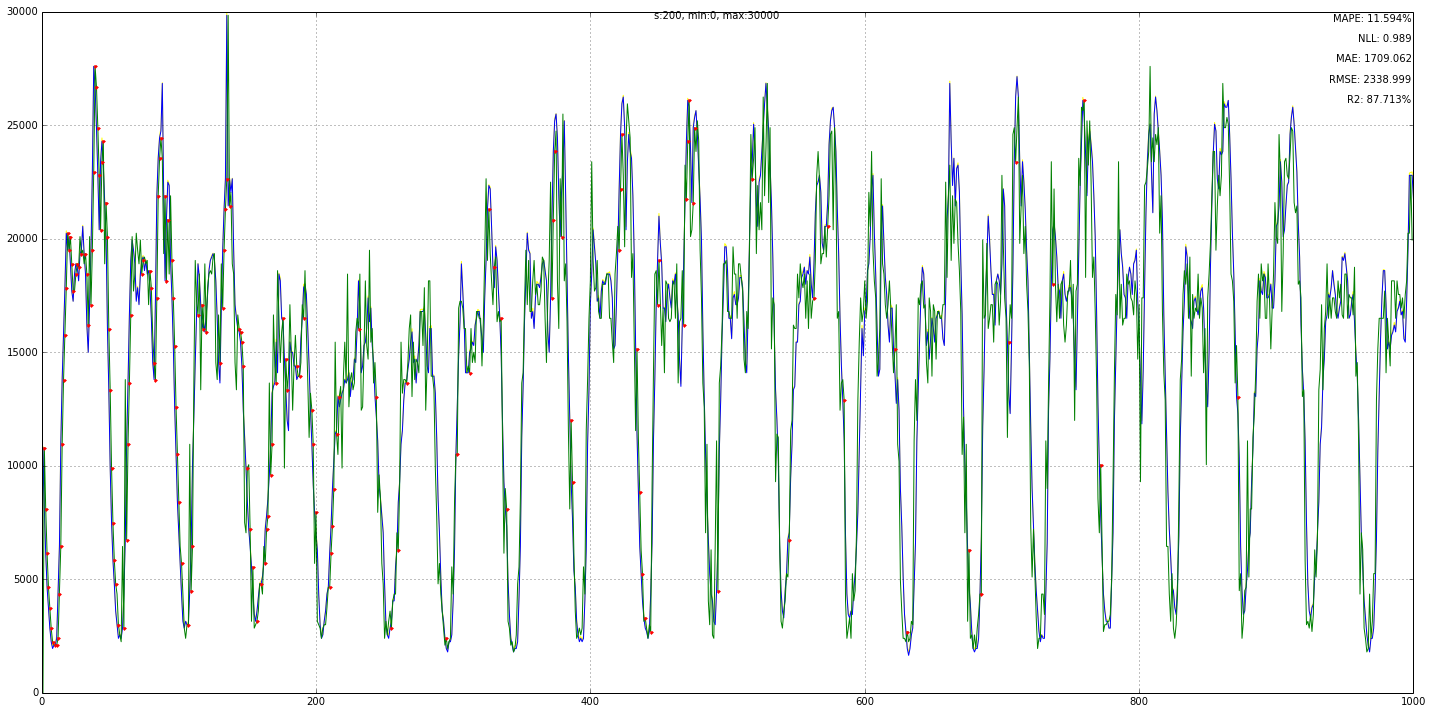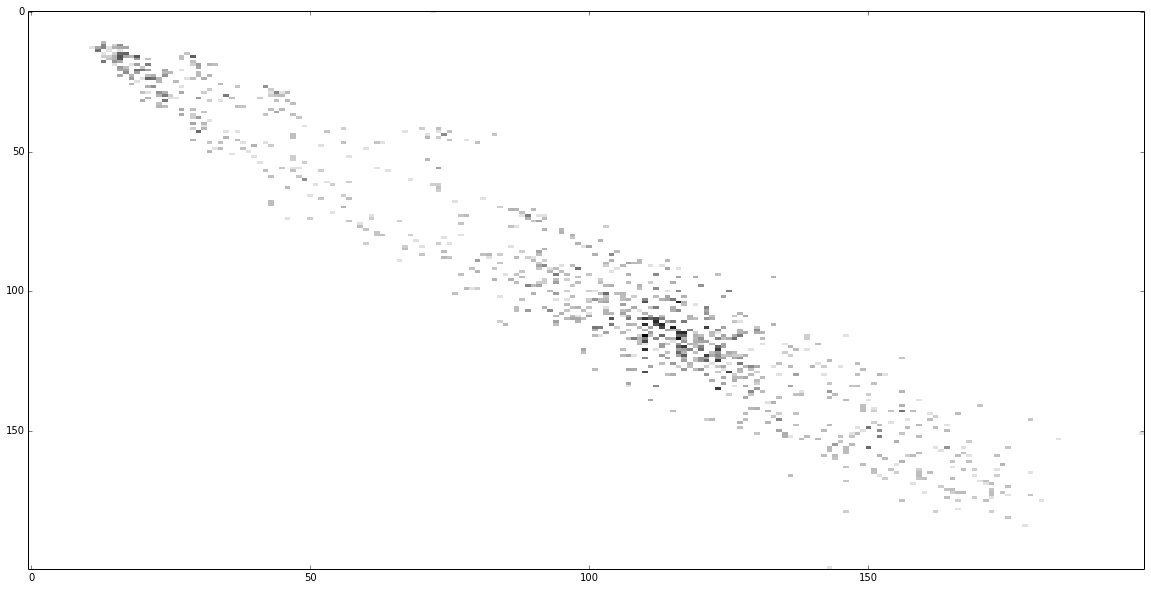DEW Moving Average¶

I had hard time to wrap my head around Reinforcement learning, still thinking of the possible implementations, implications and ramifications, thats why there will be alot of simplifications.

To spare you the mathematical baggage required to start with basic RL instead of using MDP, probability models and so on, I will try a different way of explaining the core of Reinforcement learning by using very simple math and some bold assumptions :). Also you will understand were the basic RL formula comes from, I hope.

For starters let me see if I can surmise what RL is in one line, ready ??

Exponentially weighted averaging of discounted future rewards.

got it ? if you do, no need to read further :), but please do ...

What is reinforcment learning¶

Basically the idea of RL is to find the best ACTION to execute given the accumulated knowledge so far. The way to do it is to do that is by using rewards and punishments to guide the algorithm towards a general goal. RL works in uncertain environment, from us is expected to have some idea how to REWARD the agent when it goes in the right direction. That is the greatest strength and weakness of RL, you have to, need, must know your rewards, most of the other requirements are more or less optional.

Rewards are expressed as a number, indicating how good or bad the action the agent took, was!. Rewards are just immediate bonus, to solve the RL problem we need to know the VALUE of an action i.e. the long term benefit of doing something.

Here is the obligatory pictorial representation :So the AGENT interacts with the ENVIRONMENT transitioning from STATE to STATE. By taking different ACTIONS the agent receives REWARDS and PUNISHMENTS (i.e. negative rewards). The agent make a decision between actions depending on the long term VALUE (of future accumulated rewards). (We would not talk about POLICIES in this article to simplify the discussion).

The question then is how do we calculate an ACTION long term VALUE, so that we can make best decision how to act ?

The most complete way would be to sum all the future rewards (expected return : Gt), from our current state for all possible paths of actions and pick the best one.

The formula for Expected return for a sequence is:

$$G_t = R_{t+1} + R_{t+2} + R_{t+3} +...$$

As you may suspect that is unfeasible from computational point of view.

Another hurdle is that if we have continuous process (T = infinity), Gt will be infinity for all paths-of-actions i.e. we can not distinguish which one is the best. The solution is to instead find the best next action to take, this way we don't have to calculate all action-paths, but just care only about near term prospects.

Our model¶

We will using time series again. Like we did in Threensitions.

In Threensitions we approximated the y-values in the series by a number of states S={s1,s2,s3, ... sn} i.e. we substitute real numbers with finite discrete values. (The lesson from our previous experiment is that in most of the cases 200-300 states are more than enough to represent the data range for the test signals we tried).

This time we would use a 2D table to represent transitions from one state to another, like in markov order-1 chain. There will be a twist on that but be patient.

The RL algorithm requires us to have ACTIONS, we will use the state transition to be our action. (In normal RL, actions can encode much richer behavior, but we will stick with this simple notion for now)

So our goal is to award the transition/action with a better score if it happens often enough. In the treensitions we used counting for that. But as we discussed there a model with a simple 2D counting table is not rich enough to represent complex time series, that's why we used 3D cube, so that the statistics can be more sparse.

Discounting¶

The twist with RL is that instead of counting we will use discounting. What does this mean ? It means we add smaller and smaller parts of every next reward, instead of simply summing all the rewards.

$$G_t = R_{t+1} + \gamma R_{t+2} + \gamma^2 R_{t+3} + \gamma^3 R_{t+4} + ....$$

here "gamma" is discount percentage (0,1] .

Why would we do that ? It provides mathematical tool to solve the infinity problem and as we will see in a moment allows us to do step by step calculation. The bigger gamma is, the more influence we receive from actions further away.

So the first trick is to use DISCOUNTING, rather than simple COUNTING.

In a normal RL the environment will award different rewards, but in our simple case we will award any successful transition/action with reward of 1.

Now what is the goal of our model ? The goal is to predict correctly what is the next state. This is what a normal transition model does too, but by using discounting we don't just look at the transition itself, but the subsequent transitions also have a say on how valuable the action is. There is one small problem with our current formula of discounting, we have to wait several steps before we apply the discounts. So let's refactore it abit :

$$G_t = R_{t+1} + \gamma R_{t+2} + \gamma^2 R_{t+3} + \gamma^3 R_{t+4} + ....$$$$G_t = R_{t+1} + \gamma ( R_{t+2} + \gamma R_{t+3} + \gamma^2 R_{t+4} + ....)$$$$G_t = R_{t+1} + \gamma G_{t+1}$$

Now that is more like it, we have to wait just one step to apply the discounted rewards. There is one additional subtlety, Gt, Gt+1 are all predictions, we will mention this again later.

Temporal difference¶

The second trick RL uses is the so called temporal difference. What does it mean ?

Like we said every transition/action has accumulated value depending of how often it is visited (counting) and how often states that follow are visited (discounting). There will be some tendency toward an average value on the long run, depending on which path-of-actions is winning.

One way to do this is apply the full reward at every step :

$$Q_t = Q_t + R_t$$

(Q is state-action score i.e. this is the value we store for every transition between state-A =to=> state-B in the table).

But this is cumulative reward, which if you remember suffers from the infinity problem.

This formula in our reward=1 scenario is equivalent to counting. In Threensitions app it would overflow much earlier i.e. 65353 (uint16 type) to be more correct. In our simple scenario we didn't have this problem, but if we had continuous signal, eventually we would reach this limit and flip.

What if instead we adjust it slightly towards an average Q value, like this :

$$Q_t = Q_t + \alpha * (R_t - Q_t)$$

alpha is the learning rate and describe how much of the difference between the current Q-value (the one in the table) and current Reward is applied. If the rewards trend up/down the Q will slowly follow it.

What is left now is to plug in the place of the absolute Reward the Discounted reward we mentioned earlier i.e..

$$G_t = R_{t+1} + \gamma G_{t+1}$$

So let substitute :

$$Q_t = Q_t + \alpha * (G_t - Q_t)$$$$Q_t = Q_t + \alpha * (R_{t+1} + \gamma G_{t+1} - Q_t)$$

So now the state-action (Q-value) trends toward the discounted reward.

Did you see the something ikky ... yep, when we write the algorithm we have to wait one step (t+1) before we do the calculation and then apply it backward to the previous state in the table i.e. the update happens at time t+1, rather than time t and we update Q(st, st+1) transition.

Also we use the predicted discounted reward because we don't have the real discounted reward. In the table we store the temporary value that tends toward the real value.

So how to we get the prediction, then! ... easy :

$$G \sim max(Q)$$

let's substitute :

$$Q_t = Q_t + \alpha * (R_{t+1} + \gamma * max(Q_{t+1}) - Q_t)$$

shorter for programmers :

$$Q_t \mathrel{+=} \alpha * (R_{t+1} + \gamma * max(Q_{t+1}) - Q_t)$$

The max() in the formula is the prediction part i.e. we pick the biggest Q-value between all subsequent states. That is the most probable direction the signal will go according to information we currently have.

You can visualize it like this : Every row in the table specifies the Q-value between state and the next state. Whichever column have the highest Q-value is the next predicted state (number).

Let's rewrite the formula with states included, so that you can see what I just explained :

$$Q_t(s_{t}, s_{t+1}) = Q_t(s_{t}, s_{t+1}) + \alpha * ( R_{t+1}(s_{t}, s_{t+1}) + \gamma * max(Q_{t+1}(s_{t+1}) ) - Q_t(s_{t}, s_{t+1}))$$

or in our case where the reward is always ONE, so :

$$Q_t(s_{t}, s_{t+1}) = Q_t(s_{t}, s_{t+1}) + \alpha * (1 + \gamma * max( Q_{t+1}(s_{t+1})) - Q_t(s_{t}, s_{t+1}) )$$

Exponential weighted average¶

I want to point your attention to one more thing. There is second way to interpret the Temporal difference mechanism.

If you look at the formula :

$$Q_t = Q_t + \alpha (R_t - Q_t)$$$$Q_t = Q_t + \alpha R_t - \alpha Q_t$$$$Q_t = (1 - \alpha) Q_t + \alpha * R_t$$

Is exactly the same as the formula of Exponentially weighted moving average. Now the heading of this article make sense.

DEW = Discounted Exponentially weighted : Moving average

Implementation¶

Now that we have the model let see the implementation.

It is split in two parts QLearn which is implementation of QLearn algorithm, the formula we saw above.

Then we have the wrapper around it which is the DEW average module which is mostly graphing, stats and handling of the data.

Here is how to use it :

In :
import matplotlib.pyplot as plt
%matplotlib inline
plt.rcParams['figure.figsize'] = (20.0, 10.0)

import sys
sys.path.append('../lib')
from dew_avg import *

#those DataSets are not available in the repo, it is external library and will take me some time to integrate it
# ... for now what you need is to load your data-set in 1D numpy array by some other means. Sorry..
from data_sources.data_sets import DataSet
ny_taxi = DataSet(fname='../data/nyc_taxi.csv', field='passenger_count')
In :
ny_taxi.data[:1000].max()
Out:
29993
In :
dew = DEWAvg(nstates=200, vmin=0, vmax=30000, learn_rate=0.5, gamma=0.9)
In :
dew.batch_train(ny_taxi.data[:1000], log=False)

You can look at Threensitions, for more info on stats and graphing explanation.

In :
dew.plot(nope=True)
==== MODEL stats ==============
mape: 11.594%
mae : 1709.062
rmse: 2338.999
r2: 87.713%
nll:  0.989
resolution: 150.0We can also print the Q-table, darker colors means higher value.

In :
dew.ql.qmap.max()
Out:
2.4380155439116087
In :
dew.ql.show_map()Remarks¶

So the basic idea of RL is temporal defference learning of discounted rewards.

That's all folks .....

In [ ]: